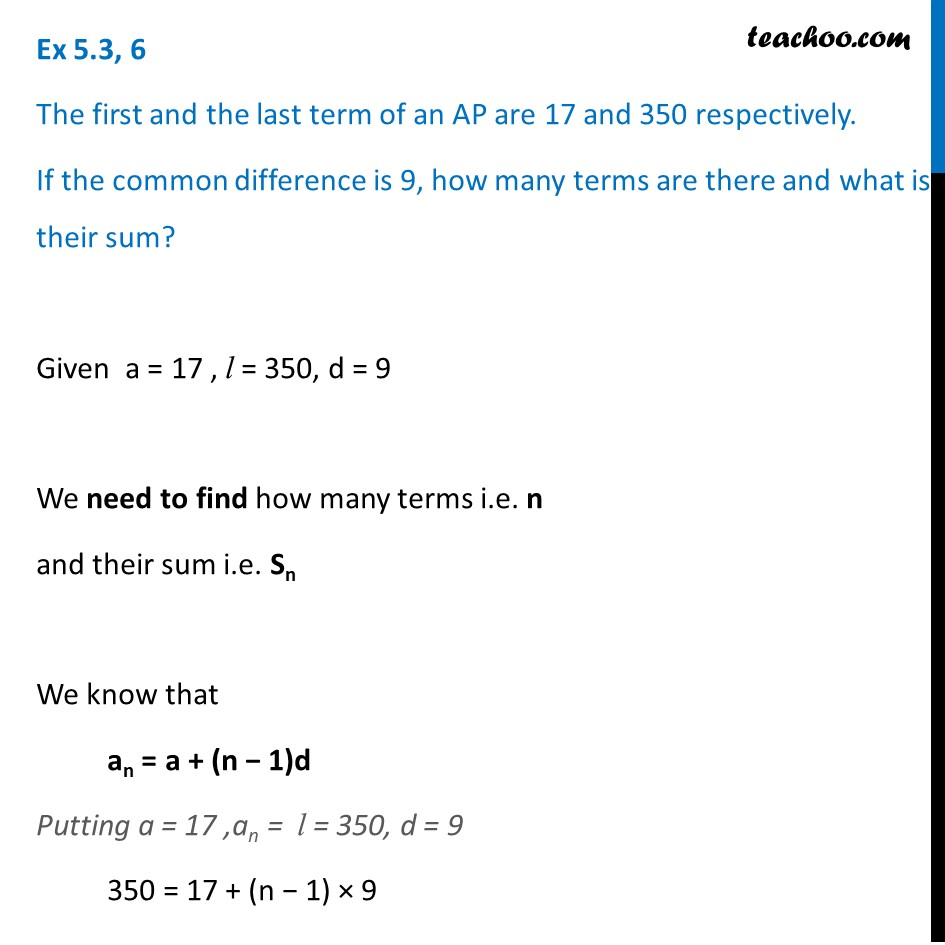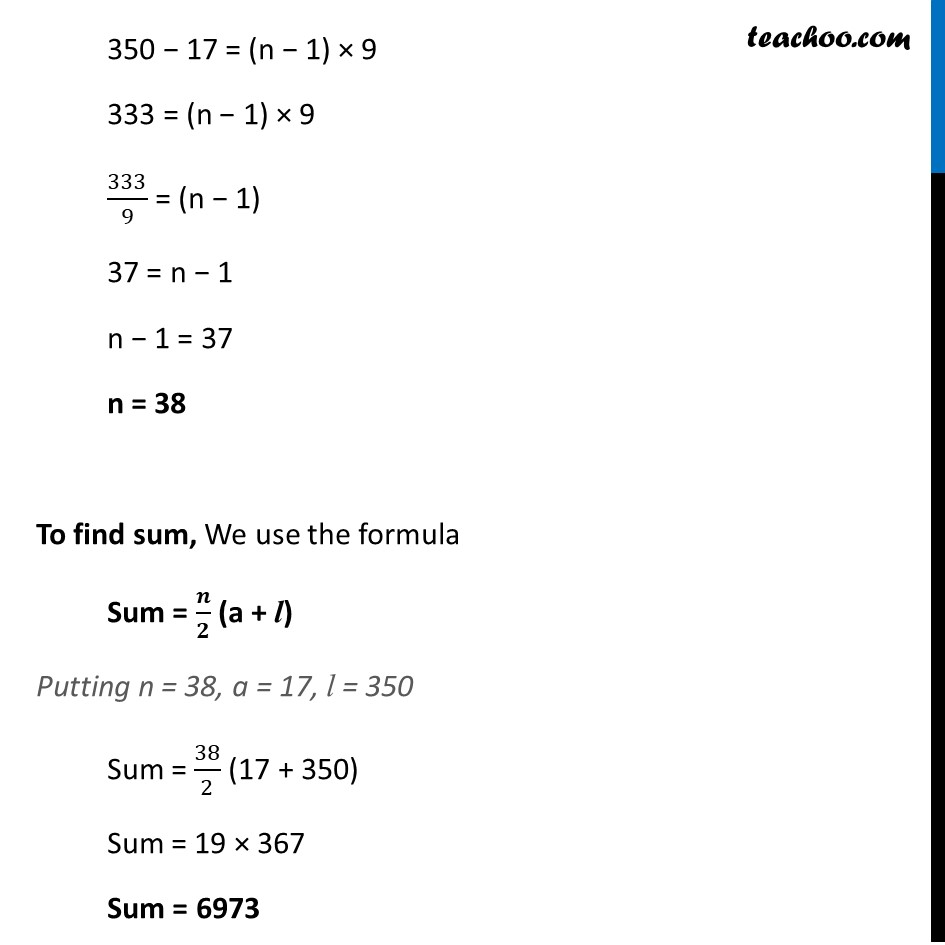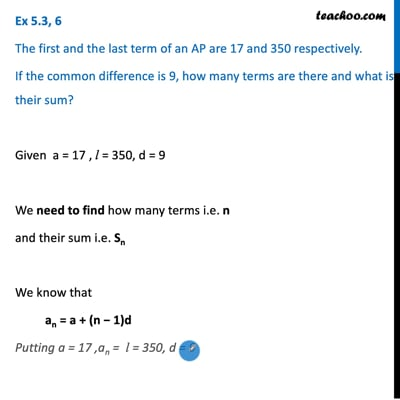Determining AP and finding sum

Chapter 5 Class 10 Arithmetic Progressions (Term 2)
Concept wiseThis video is only available for Teachoo black users

### Transcript

Ex 5.3, 6 The first and the last term of an AP are 17 and 350 respectively. If the common difference is 9, how many terms are there and what is their sum? Given a = 17 , l = 350, d = 9 We need to find how many terms i.e. n and their sum i.e. Sn We know that an = a + (n − 1)d Putting a = 17 ,an = l = 350, d = 9 350 = 17 + (n − 1) × 9 350 − 17 = (n − 1) × 9 333 = (n − 1) × 9 333/9 = (n − 1) 37 = n − 1 n − 1 = 37 n = 38 To find sum, We use the formula Sum = 𝒏/𝟐 (a + l) Putting n = 38, a = 17, l = 350 Sum = 38/2 (17 + 350) Sum = 19 × 367 Sum = 6973